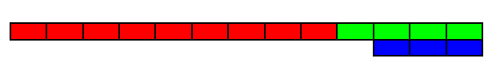#### You may also like### Prompt Cards

These two group activities use mathematical reasoning - one is numerical, one geometric.### Consecutive Numbers

An investigation involving adding and subtracting sets of consecutive numbers. Lots to find out, lots to explore.### Exploring Wild & Wonderful Number Patterns

EWWNP means Exploring Wild and Wonderful Number Patterns Created by Yourself! Investigate what happens if we create number patterns using some simple rules.

# Rod Measures

## Rod Measures

Using 3 rods of integer lengths, none longer than 10 units and not using any rod more than once, you can measure all the lengths in whole units from 1 to 10 units. How many ways can you do this?For example with rods of lengths $3, 4,$ and $9$ the measurements are:

$4-3,$ $9-4-3,$ $3,$ $4,$ $9-3,$ $9-4,$ $3+4,$ $9+3-4,$ $9,$ $9+4-3,$

Using 3 rods of ANY integer lengths, what is the greatest length N for which you can measure all lengths from 1 to N units inclusive? Can you beat 10 units? Can you beat the highest value of N submitted to date?

### Why do this problem?

This excellent problem is so very good for number awareness, and reinforcement of addition and subtraction rules.

### Possible approach

Starting off in a very practical way with suitable rods would be ideal in many circumstances.

### Key questions

How did you get to this solution?
I see you've not got a (suppose - 9 when using 2,3 & 5) can you explain that?